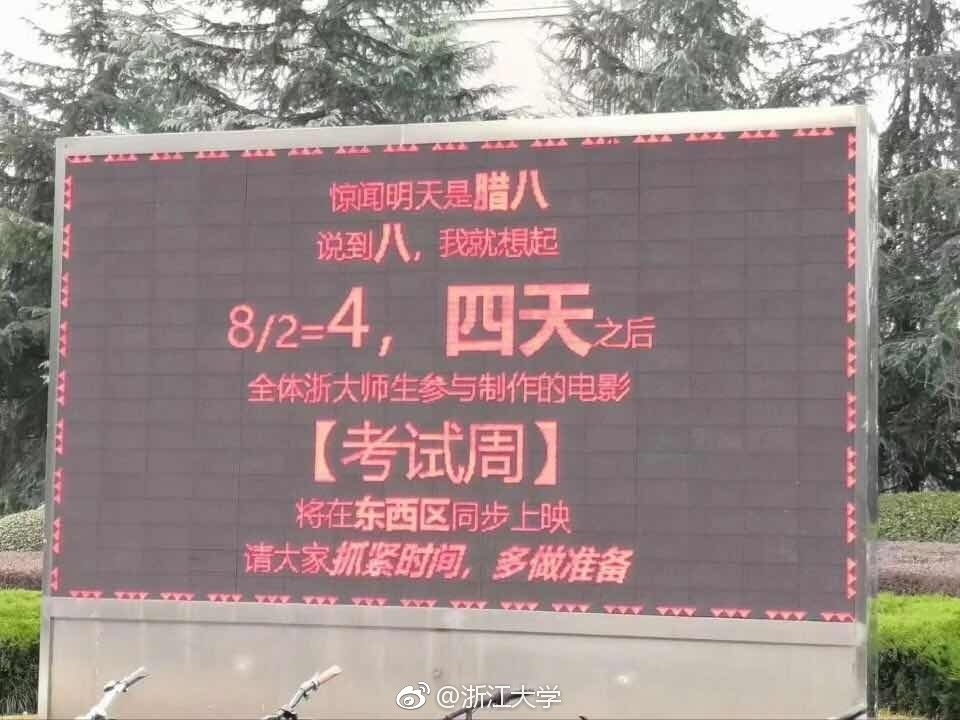# PTA 520 钻石争霸赛

## 7-1 考试周 (5分)考试周快到了，浙江大学的电子屏又调皮了…… 本题请你帮小编写一个自动倒计时的程序，对给定的日期（例如“腊八”就对应 8）和倒计时天数（例如电子屏上的“四天之后”就对应 4），自动调整公式里的分母（例如 8/2=4 里面的那个 2）。

8 3

8/2.7=3

#include <bits/stdc++.h>
using namespace std;

int main()
{
double a,b;
cin>>a>>b;
printf("%0.0f",a);
printf("/");
printf("%0.1f",a/b);
printf("=");
printf("%0.0f",b);
return 0;
}


## 7-2 真的恭喜你 (10分)

95

gong xi ni kao le 95 fen!

89

kao le 89 fen bie xie qi!

#include <bits/stdc++.h>
using namespace std;
int main(){
int a;
cin>>a;
if(a>=90&&a<=100){
cout<<"gong xi ni kao le "<<a<<" fen!"<<endl;
}
else{
cout<<"kao le "<<a<<" fen bie xie qi!"<<endl;
}
return 0;
}


## 7-3 平均成绩 (10分)

5
1 97
0 91
0 98
1 95
1 90

94.2 94.0 94.5

5
0 97
0 91
0 98
0 95
0 90

94.2 X 94.2

#include <bits/stdc++.h>
using namespace std;

int main(){
int n,s1=0,s2=0;
cin>>n;
double c=0,c1=0,c2=0;
for(int i=0;i<n;i++){
int s,g;
cin>>s>>g;
if(s==1){
c1+=g;
s1++;
}
if(s==0){
c2+=g;
s2++;
}
c=c1+c2;
}
printf("%0.1f",c/n);
if(s1==0){
printf(" X");
}else{
printf(" %0.1f",c1/s1);
}
if(s2==0){
printf(" X");
}else{
printf(" %0.1f",c2/s2);
}
}


## 7-4 古风A+B (15分)

-6666 233

-
6
4
3
3

#include <bits/stdc++.h>
#include <string>
using namespace std;

int main(){
int a,b,c;
cin>>a>>b;
c=a+b;
string s=to_string(c);
int n=s.length();
cout<<s;
for(int i=1;i<n;i++){
cout<<endl<<s[i];
}
}


## 7-5 猜近似数字 (15分)

12345678909876
2345678909876
12345678900876
12345678809876
12345678909888
1234567890987
-1

No
No
Yes
No
No

#include <bits/stdc++.h>
#include <string>
using namespace std;

int main(){
string s;
cin>>s;
int sum=s.length();
while(1){
string a;
cin>>a;
if(a=="-1") break;
int asum=a.length();
if(sum!=asum){
cout<<"No"<<endl;
continue;
}
if(sum==asum){
int count1=0;
int count2=0;
for(int i=0;i<sum;i++){
if(s[i]!=a[i]){
count1++;
if(count1>1){
break;
}
if(abs(s[i]-a[i])==1){
count2++;
}
}
}
if((count1==1&&count2==1)||count1==0){
cout<<"Yes"<<endl;
}else{
cout<<"No"<<endl;
}
}
}
}


05-2218605-21215
05-20132
05-22491
05-22176
05-201785
05-2373
05-20535
05-21237